inerton theory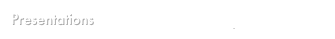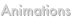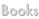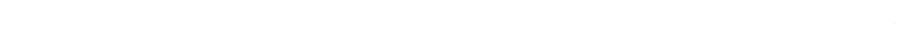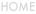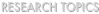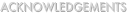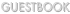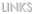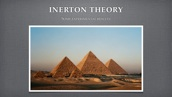presentationsThis presentation is meant as a first introduction to inerton theory. Certain mathematical concepts are used but no formulae are used in the presentation. This presentation is recommended for everybody who is new to inerton theory including scientists. It will provide a useful base for the further study of inerton theory. To access this presentation directly, click here.

In this presentation inerton theory is explained in a more scientific way, touching on the mathematical concepts and tools used to build the inerton model of real space. It is meant as a further introduction to inerton theory for those people that do not shy away from mathematics. Be aware that the mathematical concepts used are less common and that further study may be needed to fully understand the mathematical framework. To access this presentation directly, click here.

This presentation introduces a number of experimental results of inerton theory. The experiments mainly deal with phenomena hitherto never explained by science. Inerton theory tries to explain these phenomena and tries to put them in a broader spectrum. To access this presentation directly, click here.

You can click the images to go to the presentations